# Machine Learning Using Python (MEAFA Workshop)

## Lesson 6: Boosting

Boosting is one the most powerful learning algorithms developed in recent decades. In most settings, a carefully tuned boosting model is likely to be among the best algorithms immediately available for prediction, if not the best. A large scale empirical study by Caruana and Niculescu-Mizil (2006) found that boosting was overall the most accurate algorithm for supervised learning across a variety of datasets, among competitors that included random forests, support vector machines, and neural networks. Boosting is also behind the winning solutions to several machine learning competitions.

In this lesson we will study a regression problem to illustrate how to using boosting with Python. Due to the importance of this topic, we will move beyond Scikit-Learn to consider two specialised packages for gradient boosting: XGBoost and LightGBM.

This notebook relies on the following libraries and settings.

In :
# Packages
import numpy as np
from scipy import stats
import pandas as pd
import matplotlib.pyplot as plt
import seaborn as sns
import warnings
warnings.filterwarnings('ignore')

In :
# Plot settings
sns.set_context('notebook')
sns.set_style('ticks')
colours = ['#1F77B4', '#FF7F0E', '#2CA02C', '#DB2728', '#9467BD', '#8C564B', '#E377C2','#7F7F7F', '#BCBD22', '#17BECF']
crayon = ['#4E79A7','#F28E2C','#E15759','#76B7B2','#59A14F', '#EDC949','#AF7AA1','#FF9DA7','#9C755F','#BAB0AB']
sns.set_palette(colours)
%matplotlib inline
plt.rcParams['figure.figsize'] = (9, 6)

In :
# Methods
from sklearn.linear_model import LinearRegression

# Model selection and evaluation tools
from sklearn.model_selection import train_test_split
from sklearn.model_selection import RandomizedSearchCV
from sklearn.metrics import mean_squared_error, r2_score,  mean_absolute_error


## California Housing Data¶

### Data Processing¶

We use the California housing dataset, which we can obtain directly from the Scikit-Learn distribution.

In :
from sklearn.datasets import fetch_california_housing
raw = fetch_california_housing()
print(raw.DESCR)

Downloading Cal. housing from https://ndownloader.figshare.com/files/5976036 to C:\Users\Marcel\scikit_learn_data

California housing dataset.

The original database is available from StatLib

http://lib.stat.cmu.edu/datasets/

The data contains 20,640 observations on 9 variables.

This dataset contains the average house value as target variable
and the following input variables (features): average income,
housing average age, average rooms, average bedrooms, population,
average occupation, latitude, and longitude in that order.

References
----------

Pace, R. Kelley and Ronald Barry, Sparse Spatial Autoregressions,
Statistics and Probability Letters, 33 (1997) 291-297.



I prefer to work with pandas, so that the next cells stores the dataset in a dataframe.

In :
data = pd.DataFrame(raw.data, columns=raw.feature_names)
data['MedianHouseValue']=raw.target

Out:
MedInc HouseAge AveRooms AveBedrms Population AveOccup Latitude Longitude MedianHouseValue
0 8.3252 41.0 6.984127 1.023810 322.0 2.555556 37.88 -122.23 4.526
1 8.3014 21.0 6.238137 0.971880 2401.0 2.109842 37.86 -122.22 3.585
2 7.2574 52.0 8.288136 1.073446 496.0 2.802260 37.85 -122.24 3.521
3 5.6431 52.0 5.817352 1.073059 558.0 2.547945 37.85 -122.25 3.413
4 3.8462 52.0 6.281853 1.081081 565.0 2.181467 37.85 -122.25 3.422

Some of the predictors have severe outliers, which we remove for simplicity.

In :
data=data[data['AveRooms']<data['AveRooms'].quantile(.99)]
data=data[data['Population']<data['Population'].quantile(.99)]
data=data[data['AveOccup']<data['AveOccup'].quantile(.99)]


### Data Splitting¶

The following cell identifies the variables and splits the data into training and test samples. Note that:

i. The training set is only a small fraction of the data. This is to reduce the running times during our session, since the original dataset is large (20,640 observations) and methods such as random forests and boosting are relatively computationally intensitive.

ii. The response is very right skewed, so that we work with work with its log transformation.

In :
response = data.columns[-1] # last column in the dataframe
predictors= list(data.columns[:-1]) # all columns except the last

index_train, index_test  = train_test_split(np.array(data.index), train_size=0.2, random_state=1)

train = data.loc[index_train,:].copy()
test =  data.loc[index_test,:].copy()

y_train = np.log(train[response])
y_test = np.log(test[response])

X_train = train[predictors].copy()
X_test = test[predictors].copy()


## Exploratory data analysis¶

A key feature of this dataset is the presence of geographical information. The next cell draws a map to allow us to visualise the relationship between location and median house prices. Warmer colours indicate higher prices, while the size of the bubbles indicates the population of the area.

The house prices are higher near the coast, around the Bay area, and around Los Angeles. As we will see, the geographical patterns will be crucial for predictive accurarcy.

Note the following technical details:

(a) You need to install the basemap package to run the cell and render the map, with additional installation required for the full resolution option (you can remove it).

(b) The cell will take nearly a minute to run because of the full resolution option.

In :
from mpl_toolkits.basemap import Basemap

def california_map(ax=None, lllat=31.5, urlat=42.5,
lllon=-124,urlon=-113):
# This function is based on "Data Analytics Using Open-Source Tools" by Jeffrey Strickland

m = Basemap(ax=ax, projection='stere',
lon_0=(urlon + lllon) / 2,
lat_0=(urlat + lllat) / 2,
llcrnrlat=lllat, urcrnrlat=urlat,
llcrnrlon=lllon, urcrnrlon=urlon, resolution='f')
m.drawstates()
m.drawcountries()
m.drawcoastlines(color='lightblue')
return m

# Plot Figure
fig, ax = plt.subplots(figsize=(9,9))
m = california_map()
x, y = m(train['Longitude'].as_matrix(), train['Latitude'].as_matrix())

cmap = sns.diverging_palette(220, 10, as_cmap=True)
m.scatter(x,y,s=train['Population']/30, c=train['MedianHouseValue'], edgecolors='none', cmap=plt.get_cmap('rainbow'),
alpha=0.5)

ax.set_title('Median house values in California (training data)', fontsize=17, y=1.01, fontweight='bold')
ax.spines['bottom'].set_color('#DDDDDD')
ax.spines['top'].set_color('#DDDDDD')
ax.spines['right'].set_color('#DDDDDD')
ax.spines['left'].set_color('#DDDDDD')

plt.tight_layout()
plt.show()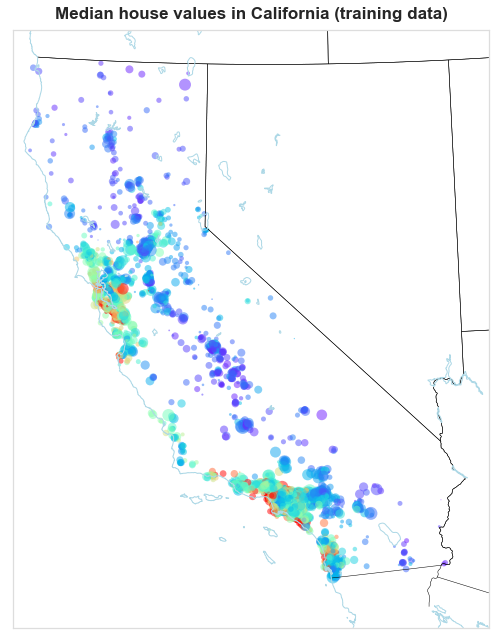The two variables with strongest linear relationship with house values are the median income of the area, and the average number of rooms. Further exploration through the scatter plots below reveal nonlinear patterns for the median income, average rooms, and average occupancy. Population, housing average age, and average bedrooms seem to have only weak relationships with house values.

In :
train.corr().round(3)

Out:
MedInc HouseAge AveRooms AveBedrms Population AveOccup Latitude Longitude MedianHouseValue
MedInc 1.000 -0.115 0.676 -0.165 -0.009 -0.080 -0.082 -0.015 0.694
HouseAge -0.115 1.000 -0.211 -0.111 -0.318 -0.014 0.013 -0.112 0.125
AveRooms 0.676 -0.211 1.000 0.259 -0.078 -0.028 0.132 -0.078 0.324
AveBedrms -0.165 -0.111 0.259 1.000 -0.056 -0.110 0.086 0.001 -0.106
Population -0.009 -0.318 -0.078 -0.056 1.000 0.190 -0.127 0.118 -0.036
AveOccup -0.080 -0.014 -0.028 -0.110 0.190 1.000 -0.175 0.184 -0.298
Latitude -0.082 0.013 0.132 0.086 -0.127 -0.175 1.000 -0.925 -0.150
Longitude -0.015 -0.112 -0.078 0.001 0.118 0.184 -0.925 1.000 -0.043
MedianHouseValue 0.694 0.125 0.324 -0.106 -0.036 -0.298 -0.150 -0.043 1.000
In :
with sns.color_palette(crayon):

fig, axes = plt.subplots(2,3, figsize=(12,8))

for i, ax in enumerate(fig.axes):
sns.regplot(X_train.iloc[:,i], y_train, scatter_kws = {'s': 15, 'alpha': 0.5}, ax=ax, fit_reg=False)
ax.set_ylabel('Log median house value')

ax.set_xlim(1, 6) # fixes a bug in the last plot

sns.despine()
plt.tight_layout()
plt.show()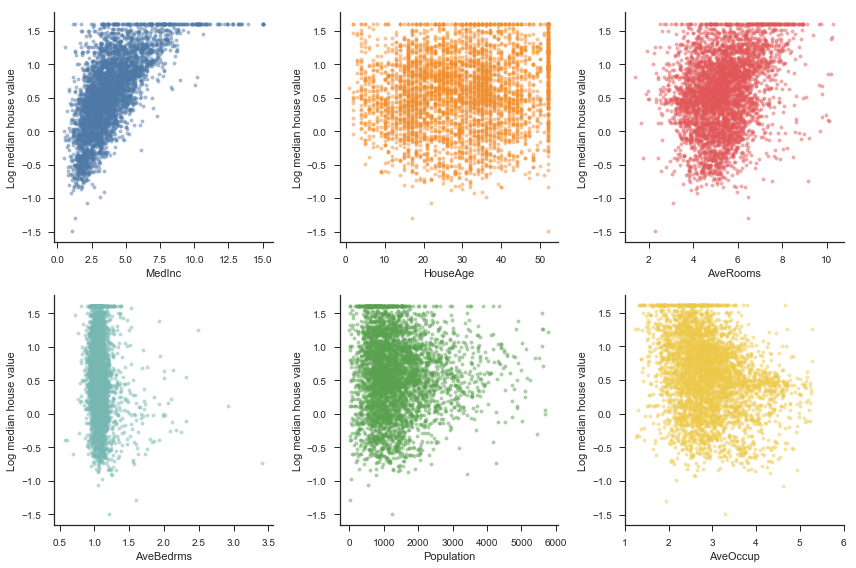## Boosting¶

We start with the Sciki-Learn implementation of boosting available in the GradientBoostingRegressor class. Recall that boosting has three crucial tuning parameters:

• The learning rate.
• The number of trees.
• The size of each tree.
• In addition, we may want to use stochastic gradient boosting by fitting each tree based on a subsample of the training data.

The basic syntax for fitting a gradient boosting regressor with Scikit-Learn is as follows.

In :
from sklearn.ensemble import GradientBoostingRegressor

gb = GradientBoostingRegressor(learning_rate= 0.05, max_depth = 4, n_estimators= 750, subsample = 1.0)
gb.fit(X_train, y_train)

Out:
GradientBoostingRegressor(alpha=0.9, criterion='friedman_mse', init=None,
learning_rate=0.05, loss='ls', max_depth=4, max_features=None,
max_leaf_nodes=None, min_impurity_decrease=0.0,
min_impurity_split=None, min_samples_leaf=1,
min_samples_split=2, min_weight_fraction_leaf=0.0,
n_estimators=750, presort='auto', random_state=None,
subsample=1.0, verbose=0, warm_start=False)

We use a randomised search to tune the model..Note that it useful to keep track of the running time, as the presence of multiple tuning parameters can make this process slow.

In :
%%time

tuning_parameters = {
'learning_rate': [0.01, 0.05, 0.1],
'n_estimators' : [250, 500, 750, 1000, 1500, 2000],
'max_depth' : [2 ,3, 4],
'subsample' : [0.6, 0.8, 1.0]
}

# Using GridSearchCV would be too slow. Increase the number of iterations to explore more hyperparameter combinations.
gb = RandomizedSearchCV(model, tuning_parameters, n_iter = 20, cv = 10, return_train_score=False, n_jobs=4)
gb.fit(X_train, y_train)

print('Best parameters found by randomised search:', gb.best_params_, '\n')

Best parameters found by randomised search: {'subsample': 0.8, 'n_estimators': 750, 'max_depth': 4, 'learning_rate': 0.05}

Wall time: 4min 17s

In :
gb.best_estimator_

Out:
GradientBoostingRegressor(alpha=0.9, criterion='friedman_mse', init=None,
learning_rate=0.05, loss='ls', max_depth=4, max_features=None,
max_leaf_nodes=None, min_impurity_decrease=0.0,
min_impurity_split=None, min_samples_leaf=1,
min_samples_split=2, min_weight_fraction_leaf=0.0,
n_estimators=750, presort='auto', random_state=None,
subsample=0.8, verbose=0, warm_start=False)

I have separtely coded a function to plot the importance of the variables.

In :
from statlearning import plot_feature_importance

plot_feature_importance(gb.best_estimator_, predictors)
plt.show()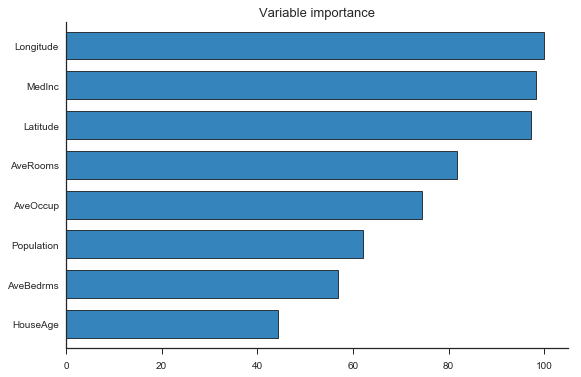## XGBoost¶

XGBoost is a state-of-art gradient boosting library that is very popular among Kaggle users. The easiest way to get started with XGBoost is to use the Scikit-Learn API provided by the package. That makes the syntax identical to what we did above, except that we call the XGBRegressor class from the XGBoost package.

In :
import xgboost as xgb

bst = xgb.XGBRegressor(learning_rate= 0.05, max_depth = 4, n_estimators= 750, subsample = 1.0)
bst.fit(X_train, y_train)

Out:
XGBRegressor(base_score=0.5, booster='gbtree', colsample_bylevel=1,
colsample_bytree=1, gamma=0, learning_rate=0.05, max_delta_step=0,
max_depth=4, min_child_weight=1, missing=None, n_estimators=750,
n_jobs=1, nthread=None, objective='reg:linear', random_state=0,
reg_alpha=0, reg_lambda=1, scale_pos_weight=1, seed=None,
silent=True, subsample=1.0)
In :
%%time

model = xgb.XGBRegressor()

tuning_parameters = {
'learning_rate': [0.01, 0.05, 0.1],
'n_estimators' : [250, 500, 750, 1000, 1500, 2000],
'max_depth' : [2,3,4],
'subsample' : [0.6, 0.8, 1.0]
}

bst = RandomizedSearchCV(model, tuning_parameters, n_iter = 20, cv = 10, return_train_score=False, n_jobs=4)
bst.fit(X_train, y_train)

print('Best parameters found by randomised search:', bst.best_params_, '\n')

Best parameters found by randomised search: {'subsample': 0.6, 'n_estimators': 2000, 'max_depth': 4, 'learning_rate': 0.05}

Wall time: 4min 23s


XGBoost also supports regularisation of the tree weights (the alpha and lambda hyperparameters), even though we do not use these features here.

In :
bst.best_estimator_

Out:
XGBRegressor(base_score=0.5, booster='gbtree', colsample_bylevel=1,
colsample_bytree=1, gamma=0, learning_rate=0.05, max_delta_step=0,
max_depth=4, min_child_weight=1, missing=None, n_estimators=2000,
n_jobs=1, nthread=None, objective='reg:linear', random_state=0,
reg_alpha=0, reg_lambda=1, scale_pos_weight=1, seed=None,
silent=True, subsample=0.6)
In :
plot_feature_importance(bst.best_estimator_, labels=predictors)
plt.show()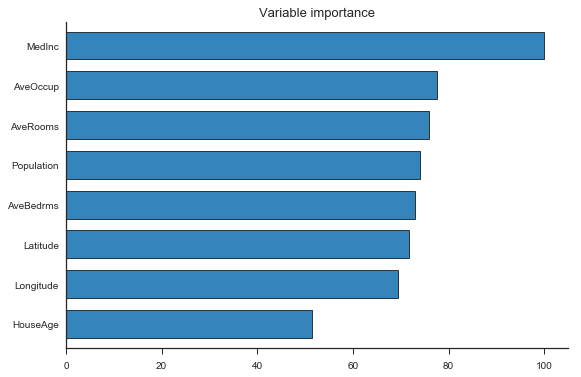The XGBoost functionately extends well beyond the Scikit-Learn API. Below, we construct a pure XGBoost implementation to select the number of boosting iterations by cross-validation and early stopping.

In :
%%time

dtrain = xgb.DMatrix(X_train, y_train) # we need to convert the data to the format required by xgboost
dtest  = xgb.DMatrix(X_test)

param = {
'max_depth': 4,
'learning_rate': 0.05,
'subsample': 0.8,
'silent' : 0,
'objective':'reg:linear',
}

cv = xgb.cv(param, dtrain, num_boost_round = 2000, nfold=10, early_stopping_rounds=50)

print(f'Selected number of boosting iterations: {cv.shape}')
print(f'RMSE (CV): {cv.iloc[-1,0]:.4f}')

Selected number of boosting iterations: 1095
RMSE (CV): 0.2218
Wall time: 1min 34s

In :
fig, ax = plt.subplots(figsize=(8,5))
plt.plot(cv.iloc[:,0])
ax.set_ylabel('Cross-validation RMSE')
ax.set_xlabel('Boosting iterations')
sns.despine()
plt.show()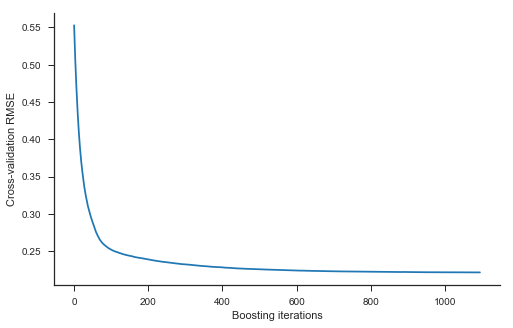## LightGBM¶

LightGBM is a gradient boosting library developed by Microsoft, and a competitor to XGBoost. Similarly to XGBoost, it provides an Scikit-Learn API that makes it simple to use. Notice how it runs much faster than the the Scikit-Learn implementation of boosting.

In :
import lightgbm as lgb

In :
%%time

model = lgb.LGBMRegressor(objective='regression')

tuning_parameters = {
'learning_rate': [0.01, 0.05, 0.1],
'n_estimators' : [250, 500, 750, 1000, 1500, 2000],
'max_depth' : [2, 3, 4],
'subsample' : [0.6, 0.8, 1.0],
}

lbst = RandomizedSearchCV(model, tuning_parameters, n_iter = 20, cv = 10, return_train_score=False, n_jobs=4)
lbst.fit(X_train, y_train)

print('Best parameters found by randomised search:', lbst.best_params_, '\n')

Best parameters found by randomised search: {'subsample': 0.8, 'n_estimators': 500, 'max_depth': 4, 'learning_rate': 0.1}

Wall time: 1min 3s


## Benchmark Models¶

### OLS¶

In :
ols = LinearRegression()
ols.fit(X_train, y_train)

Out:
LinearRegression(copy_X=True, fit_intercept=True, n_jobs=1, normalize=False)

### Regression tree¶

The basic syntax for fitting a regression tree by recursive binary splitting is the following, where we allow the tree to have a maximum depth of two for visualisation. I also specify the minimum number of samples in the terminal nodes to highlight the importance explictly controlling this tuning parameter.

In :
from sklearn.tree import DecisionTreeRegressor

tree = DecisionTreeRegressor(max_depth=2, min_samples_leaf=5)
tree.fit(X_train, y_train)

Out:
DecisionTreeRegressor(criterion='mse', max_depth=2, max_features=None,
max_leaf_nodes=None, min_impurity_decrease=0.0,
min_impurity_split=None, min_samples_leaf=5,
min_samples_split=2, min_weight_fraction_leaf=0.0,
presort=False, random_state=None, splitter='best')

To plot the decision tree, we need to use the python-graphviz package. You should experiment with letting the tree grow a few more steps and interpreting the result.

In :
from sklearn.tree import export_graphviz
import graphviz

dot_data = export_graphviz(tree, out_file=None, feature_names=predictors, impurity=False, rounded=True)
graph = graphviz.Source(dot_data)
graph.render('tree')
graph

Out:

Next, we find a tree of optimal size for prediction.

In :
# This cell may take half a minute to run

model = DecisionTreeRegressor(min_samples_leaf=5)

tuning_parameters = {
'min_samples_leaf': [1,5,10,20],
'max_depth': np.arange(1,30),
}

tree = RandomizedSearchCV(model, tuning_parameters, n_iter=20, cv=10, return_train_score=False)
tree.fit(X_train, y_train)

print('Best parameters:', tree.best_params_)

Best parameters: {'min_samples_leaf': 10, 'max_depth': 20}


### Random Forest¶

In :
from sklearn.ensemble import RandomForestRegressor

rf = RandomForestRegressor(n_estimators=2000, max_features = 3, min_samples_leaf= 1)
rf.fit(X_train, y_train)

Out:
RandomForestRegressor(bootstrap=True, criterion='mse', max_depth=None,
max_features=3, max_leaf_nodes=None, min_impurity_decrease=0.0,
min_impurity_split=None, min_samples_leaf=1,
min_samples_split=2, min_weight_fraction_leaf=0.0,
n_estimators=2000, n_jobs=1, oob_score=False, random_state=None,
verbose=0, warm_start=False)

Recall that the key tuning parameters for a random forest are (i) the number of randomly selected candidate variables for each split (b) the mininum node size. I selected the specification above with a grid search (omitted here to save time).

## Model evaluation¶

In :
columns=['Test RMSE', 'Test R2', 'Test MAE']
rows=['Linear regression', 'Decision tree', 'Random forest', 'SKLearn Boost', 'XGBoost', 'LightGBM']
results=pd.DataFrame(0.0, columns=columns, index=rows)

methods=[ols, tree, rf, gb, bst, lbst]

for i, method in enumerate(methods):

y_pred=method.predict(X_test)
results.iloc[i,0] = np.sqrt(mean_squared_error(y_test, y_pred))
results.iloc[i,1] = r2_score(y_test, y_pred)
results.iloc[i,2] = mean_absolute_error(y_test, y_pred)

results.round(3)

Out:
Test RMSE Test R2 Test MAE
Linear regression 0.326 0.674 0.247
Decision tree 0.306 0.714 0.221
Random forest 0.253 0.805 0.181
SKLearn Boost 0.235 0.830 0.165
XGBoost 0.234 0.832 0.164
LightGBM 0.236 0.829 0.167